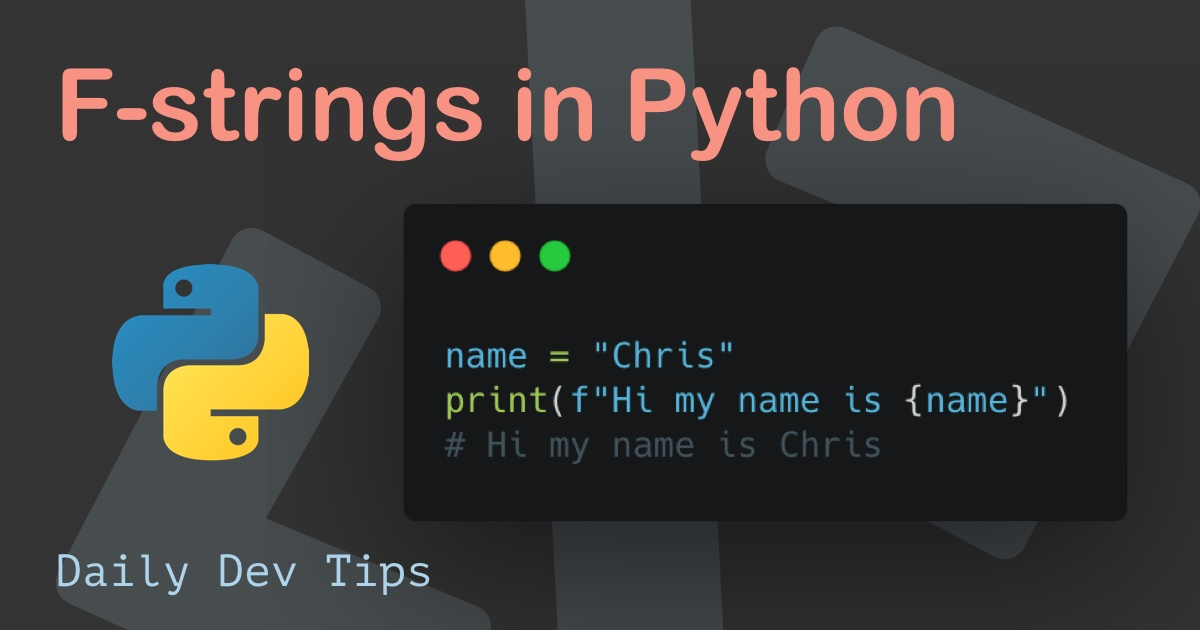Subscribe

# Python if...else statements

✍️

The basics of if else statements in Python

22 May, 2021 · 3 min read

Since I'm still new to exploring the Python world, I thought it would be good to understand the syntax behind if...else statements.

## Basic if statement in Python permalink

Let's start by looking at a regular if statement. In python this will be used as the following syntax.

``````if condition:
# do something``````

You might want to check a variable for True/False, check if a number is higher/lower, or a string is a certain value.

``````number = 5
string = "Chris"
boolean = True

if number > 3:
print("Number is positive")

if string == "Chris":
print("Chris in the building")

if boolean == True:
print("Boolean is true")``````

This will result in the following:

``````Number is positive
Chris in the building
Boolean is true``````

## Multiple returns for an if statement permalink

The cool part about this is that we can have multiple returns by using the correct indentation.

Let's say we need two lines of prints.

``````if number > 3:
print("Number is positive")
print("This is a second positive line")``````

That will return both lines if the statement is met!

As you may have guessed, this also gives us an excellent opportunity to use an else statement if the if fails.

The logic for that is as follows:

``````if condition:
# do something
else:
# do something else``````

Let's try that out with a better use case.

``````number = 10

if number > 20:
print("Number is bigger then 20")
else:
print("It's a smaller number")``````

Running this code will result in:

``It's a smaller number``

The if...else might be a good solution for static boolean checks. In most real-world examples, you might want to add a specific second, third, or more if.

For that, we can use the `elif`, which states if the previous condition was not met, try this one. This can still fall back to an `else` if we define one.

The logic:

``````if condition:
# do thing 1
elif condition 2:
# do thing 2
else:
# do something else``````

Let's try that out by checking if a number is smaller or equal.

``````a = 5
b = 5
if(a > b):
print("A is greater than B")
elif a == b:
print("A and B are equal")
else:
print("B is greater than A")``````

Which will result in:

``A and B are equal``

This kind of `elif` statement can be used multiple times.

Tweet this tip

### Speedtest your connection in Python

21 Jun, 2021 · 2 min read### F-strings in Python

7 Jun, 2021 · 2 min readJoin 2101 devs and subscribe to my newsletter

• 1000 articles written
• 2101 devs subscribed
• 529116 words written